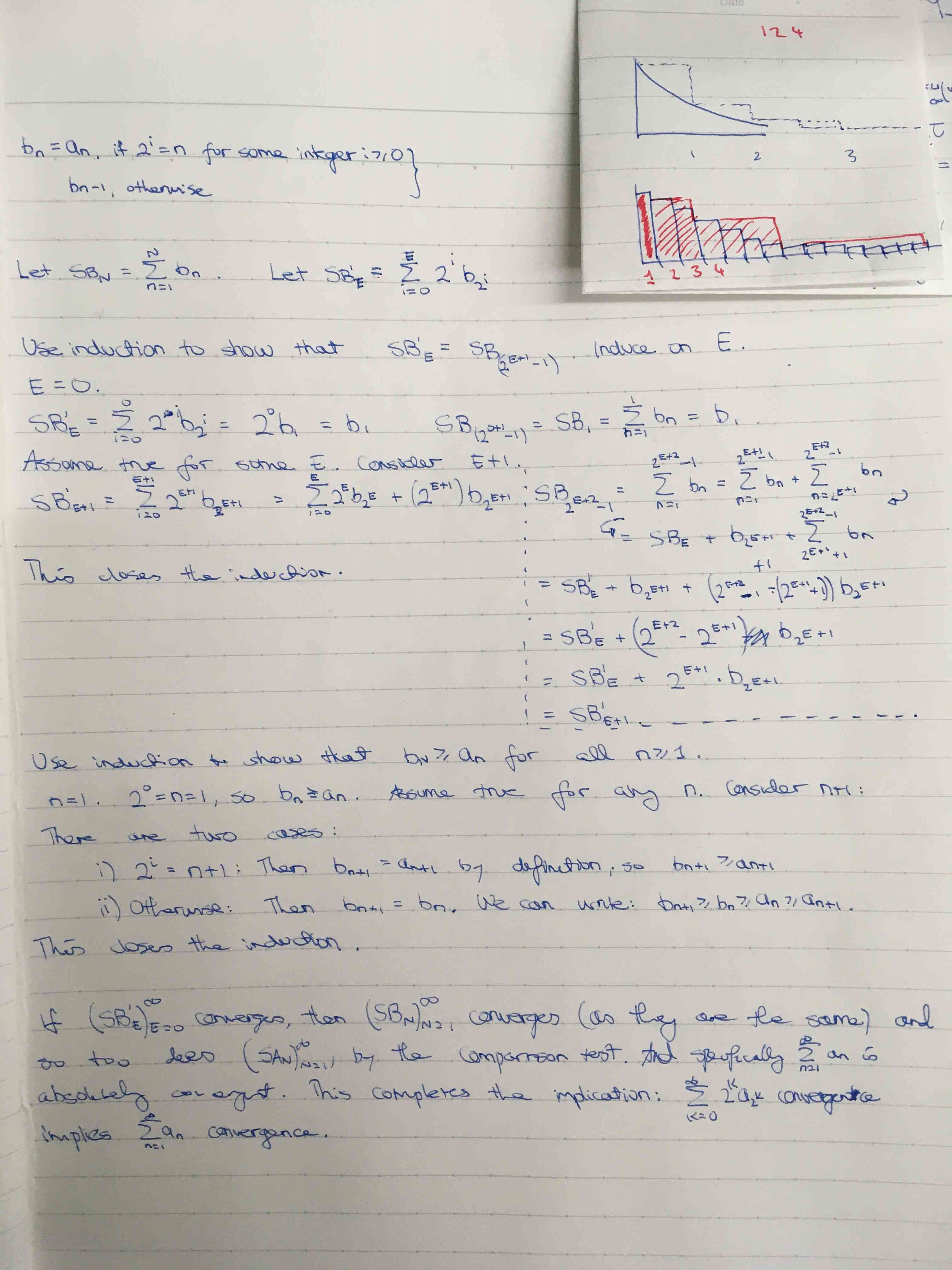Show Question
Math and science::Analysis::Tao::07. Series

# Cauchy criterion, harmonic series and the Riemann-zeta function

Let $$(a_n)_{n=1}^{\infty}$$ be a decreasing sequence of non-negative real numbers. Then the series $$\sum_{n=1}^{\infty}a_n$$ is convergent if and only if the series

$\sum_{k=0}^{\infty} 2^ka_{2^k} = a_1 + 2a_2 + 4a_4 + 8a_8 + ...$

is convergent. This is the Cauchy criterion.

### Harmonic series

The Cauchy criterion can be used to show that the Harmonic series, $$\sum_{n=1}^{\infty}\frac{1}{n}$$, is divergent. Yet $$\sum_{n=1}^{\infty}\frac{1}{n^2}$$ is convergent.

### Riemann-zeta function

The quantity $$\sum_{n=1}^{\infty}\frac{1}{q^n}$$ is called the Riemann-zeta function of q and is denoted by $$\zeta(q)$$. This function is very important in number theory in particular for investigating the distribution of primes. There is a famous unsolved problem regarding this function called the Riemann hypothesis.

An interesting feature of this criterion is that it only uses a small number of elements of the sequence $$a_n$$ (namely, those elements whose index is a power of 2, $$n = 2_k \text{ for some integer k > 0}$$) in order to deterimen whether the whole series is convergent or not.

### Intuition

There is a visual/geometric understanding that makes the mechanism of the proof clear. See the drawing below.

The Cauchy Criterion introduces the idea of bunching together segments of the sum to make a new sum, and by clever bunching we can see if the series is decreasing fast enough for the sum to converge.

I think it is correct to say that if a series diverges, then there will exist a bunching of the sum such that each bunch is greater than some constant. This idea can be seen a bit more clearly if you try and create a clever bunching for the sum $$\sum_{n=1}^{\infty}\frac{1}{n^2}$$—you will find that there is no bunching that will work to form a constant, instead the bunches will keep getting smaller.

### Proof

The proof of this is not short. The book has a more consise approach than what I took. The first half of my approach is:The choice of $$2^k$$ seems quite natural when tackling the harmonic series. Try to group the harmonic series elements as above such that they sum to 1. You end up with $$1\times \frac{1}{1}, 2\times \frac{1}{2}, 4\times \frac{1}{4}...$$. Already, this coves $$1+2+4 = 7$$ elements, so the next will be the 8th whose value is $$\frac{1}{8}$$ requiring a grouping of 8 elements, bringing us up to 15, and so on.

### Divergence of the harmonic series

The proof of the following proposition is an example of the application of the Cauchy criterion.

Let $$q > 0$$ be a rational number. Then the series $$\sum_{n=1}^{\infty} \frac{1}{n^q}$$ is convergent when $$q > 1$$ and divergent when $$q \le 1$$.

A particular divergent case of this series is the harmonic series: $$\sum_{n=1}^{\infty} \frac{1}{n}$$. In comparison, $$\sum_{n=1}^{\infty} \frac{1}{n^2}$$ is convergent.

#### Proof

The sequence $$(\frac{1}{n^q})_{n=1}^{\infty}$$ is non-negative and decreasing (by Lemma 5.6.9 d), and so the Cauchy criterion applies. Thus, the sequence is convergent if and only if

$\sum_{k=0}^{\infty}2^k \frac{1}{(2^k)^q}$

is convergent. By the laws of exponentiation we can rewrite this as

$\sum_{k=0}^{\infty}(2^{1-q})^k$

This is a geometric series ($$\sum_{k=0}^{\infty}x^k$$) and converges if and only if $$|x| < 1$$. Thus our original $$\sum_{n=1}^{\infty} \frac{1}{n^q}$$ will converge if and only if $$|2^{1-q}| < 1$$, which happens if and only if $$q > 1$$ (why?).

p174